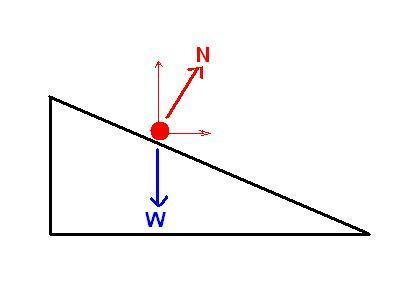# Calculating acceleration of ball rolling down ramp

xwkkx

## Homework Statement

Hi, I know the acceleration of steel ball rolling down the inclined track is 5/7 * gsin(theta). But is it possible to find the acceleration of ball rolling on the inclined track just by using the distance travelled on the horizontal plane(attached to the Inclined track) and the time taken? Assuming constant velocity and no friction when rolling on the horizontal plane.

## Homework Equations

vf^2 = vi^2 +2as -- 1
v(diagonal) = v(horizontal)/cos(theta) -- 2

## The Attempt at a Solution

As we know that the speed along the horizontal plane is constant, the velocity = distance/time. Assuming it travelled 1m in 1s, velocity = 1m/s.
final velocity when ball reaches the end of ramp = initial velocity of ball at the start of horizontal plane
thus, final velocity = v(horizontal)/ cos(theta) -- same as eq 2
with the final velocity, we can use eqn 1 to calculate the acceleration assuming we have the length of ramp.
Is this method correct? or am i missing something? this method will only work if assuming there is no loss of mechanical energy??

Homework Helper

## Homework Statement

Hi, I know the acceleration of steel ball rolling down the inclined track is 5/7 * gsin(theta). But is it possible to find the acceleration of ball rolling on the inclined track just by using the distance travelled on the horizontal plane(attached to the Inclined track) and the time taken? Assuming constant velocity and no friction when rolling on the horizontal plane.

## Homework Equations

vf^2 = vi^2 +2as -- 1
v(diagonal) = v(horizontal)/cos(theta) -- 2

## The Attempt at a Solution

As we know that the speed along the horizontal plane is constant, the velocity = distance/time. Assuming it travelled 1m in 1s, velocity = 1m/s.
final velocity when ball reaches the end of ramp = initial velocity of ball at the start of horizontal plane
thus, final velocity = v(horizontal)/ cos(theta) -- same as eq 2
with the final velocity, we can use eqn 1 to calculate the acceleration assuming we have the length of ramp.
Is this method correct? or am i missing something? this method will only work if assuming there is no loss of mechanical energy??

Welcome to PF!

Why do you think that the horizontal component of the velocity is constant?

xwkkx
i know velocity is not constant as there is friction but what if we assume there is no friction action on the ball?

Homework Helper
Assume a piece of ice sliding down along an incline. There is no friction. Is the horizontal component of velocity constant?

xwkkx
Im thinking yes. cos there is no force along the horizontal component hence velocity will be constant

Homework Helper
Im thinking yes. cos there is no force along the horizontal component hence velocity will be constant
Gravity has no horizontal component, but there is the normal force N from the incline acting also on the piece of ice, or on the rolling ball. The normal force has horizontal component.J Hann
You need to consider the moment of inertia of the ball. That's why the acceleration of a ball
would be different from that of a cylinder or a hoop.